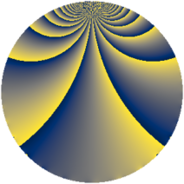# Properties

 Label 3549.2.byLevel $3549$ Weight $2$ Character orbit 3549.by Rep. character $\chi_{3549}(1441,\cdot)$ Character field $\Q(\zeta_{12})$ Dimension $824$ Sturm bound $970$

# Related objects

## Defining parameters

 Level: $$N$$ $$=$$ $$3549 = 3 \cdot 7 \cdot 13^{2}$$ Weight: $$k$$ $$=$$ $$2$$ Character orbit: $$[\chi]$$ $$=$$ 3549.by (of order $$12$$ and degree $$4$$) Character conductor: $$\operatorname{cond}(\chi)$$ $$=$$ $$91$$ Character field: $$\Q(\zeta_{12})$$ Sturm bound: $$970$$

## Dimensions

The following table gives the dimensions of various subspaces of $$M_{2}(3549, [\chi])$$.

Total New Old
Modular forms 2056 824 1232
Cusp forms 1832 824 1008
Eisenstein series 224 0 224

## Trace form

 $$824q + 2q^{7} + 412q^{9} + O(q^{10})$$ $$824q + 2q^{7} + 412q^{9} + 16q^{11} - 64q^{14} + 424q^{16} + 10q^{21} + 32q^{22} + 56q^{28} + 16q^{29} + 40q^{32} + 8q^{35} + 28q^{37} + 12q^{42} + 12q^{43} + 16q^{44} + 112q^{46} + 30q^{49} - 176q^{50} + 224q^{53} + 180q^{56} - 48q^{57} - 48q^{58} + 104q^{60} + 4q^{63} - 16q^{67} + 56q^{70} + 24q^{71} + 16q^{74} - 96q^{79} - 412q^{81} - 20q^{84} + 56q^{85} + 48q^{86} - 216q^{88} + 16q^{92} - 12q^{93} - 96q^{95} - 36q^{98} - 16q^{99} + O(q^{100})$$

## Decomposition of $$S_{2}^{\mathrm{new}}(3549, [\chi])$$ into newform subspaces

The newforms in this space have not yet been added to the LMFDB.

## Decomposition of $$S_{2}^{\mathrm{old}}(3549, [\chi])$$ into lower level spaces

$$S_{2}^{\mathrm{old}}(3549, [\chi]) \cong$$ $$S_{2}^{\mathrm{new}}(91, [\chi])$$$$^{\oplus 4}$$$$\oplus$$$$S_{2}^{\mathrm{new}}(273, [\chi])$$$$^{\oplus 2}$$$$\oplus$$$$S_{2}^{\mathrm{new}}(1183, [\chi])$$$$^{\oplus 2}$$